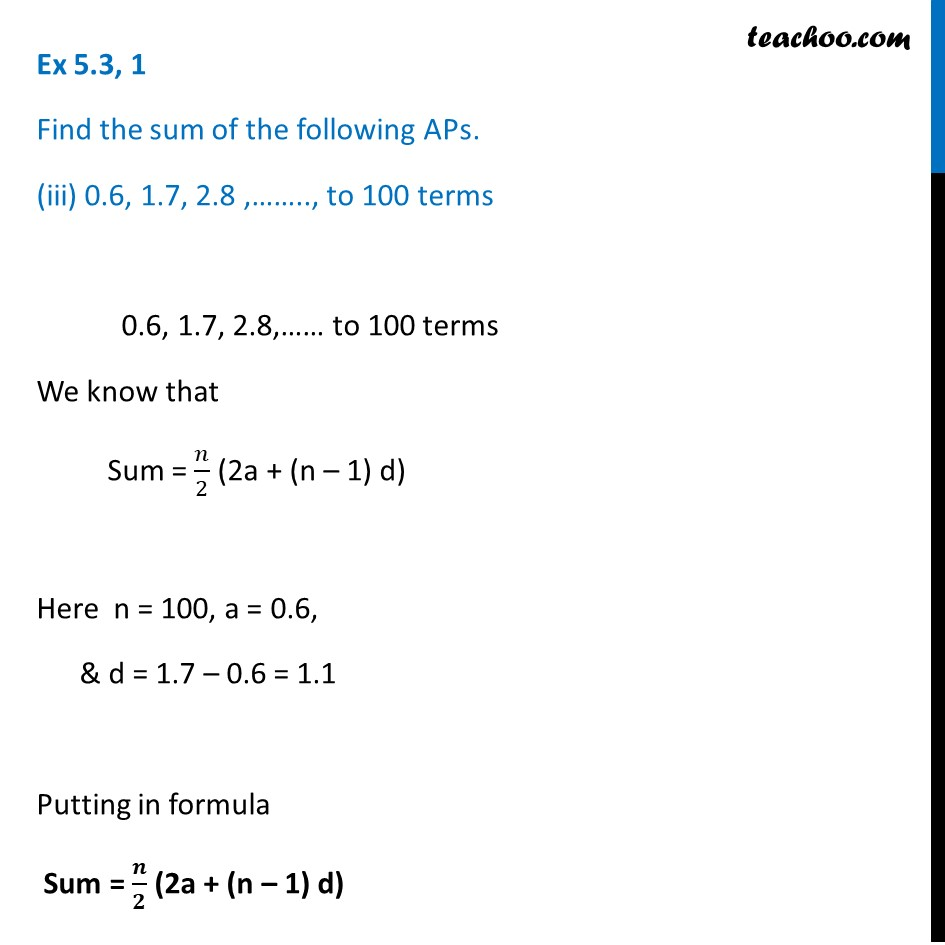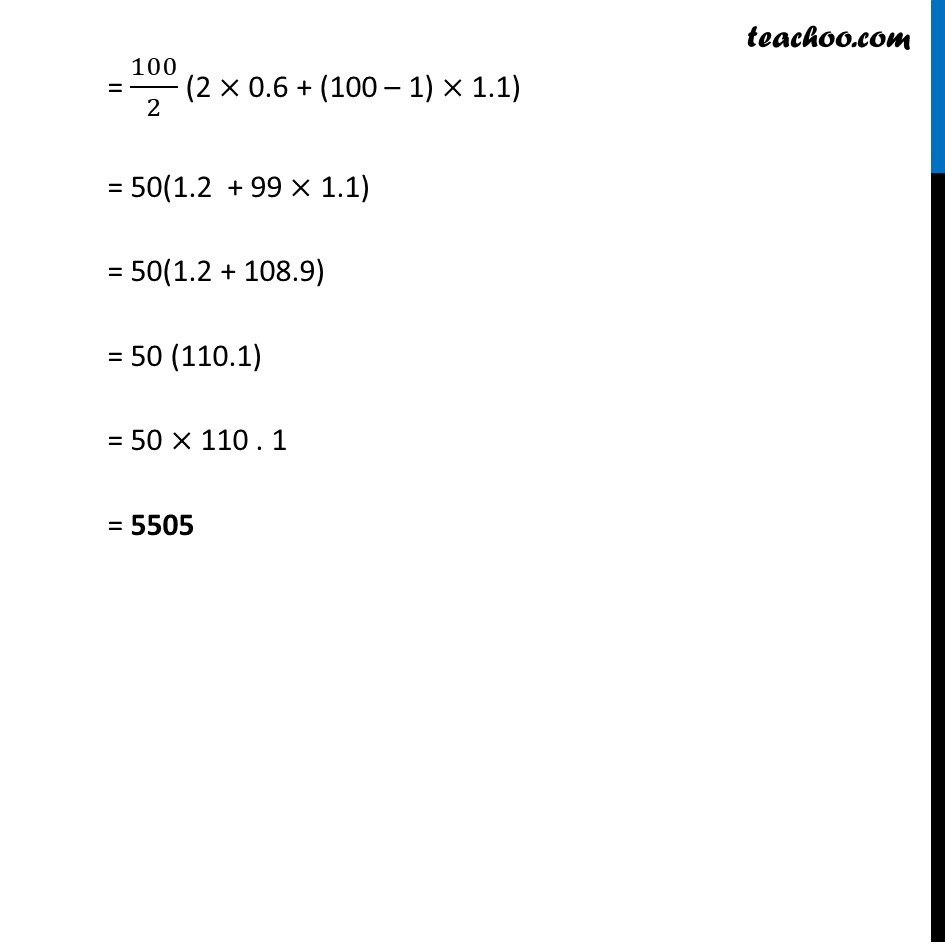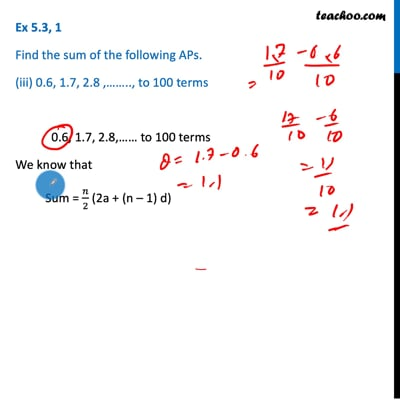Ex 5.3

Chapter 5 Class 10 Arithmetic Progressions
Serial order wiseThis video is only available for Teachoo black users

Maths Crash Course - Live lectures + all videos + Real time Doubt solving!

### Transcript

Ex 5.3, 1 Find the sum of the following APs. (iii) 0.6, 1.7, 2.8 ,…….., to 100 terms 0.6, 1.7, 2.8,…… to 100 terms We know that Sum = 𝑛/2 (2a + (n – 1) d) Here n = 100, a = 0.6, & d = 1.7 – 0.6 = 1.1 Putting in formula Sum = 𝒏/𝟐 (2a + (n – 1) d) = 100/2 (2 × 0.6 + (100 – 1) × 1.1) = 50(1.2 + 99 × 1.1) = 50(1.2 + 108.9) = 50 (110.1) = 50 × 110 . 1 = 5505